# Number train

The numbers 1,2,3,4,5,6,7,8 and 9 traveled by train. The train had three cars and each was carrying just three numbers. No. 1 rode in the first carriage, and in the last carriage was all odd numbers. The conductor calculated sum of the numbers in the first, second and last car, and each time he came out the same sum. Determine how numbers can be divided into the car. How many has this problem solutions?

n =  1

### Step-by-step explanation:

::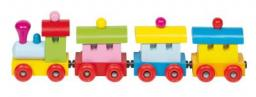Did you find an error or inaccuracy? Feel free to write us. Thank you!

Showing 1 comment:Prachij.maths@gmail.com
please give a general method for forming natural number train## Related math problems and questions:

• Z9–I–4 MO 2017Numbers 1, 2, 3, 4, 5, 6, 7, 8 and 9 were prepared for a train journey with three wagons. They wanted to sit out so that three numbers were seated in each carriage and the largest of each of the three was equal to the sum of the remaining two. The conduct
• Star equationWrite digits instead of stars so that the sum of the written digits is odd and is true equality: 42 · ∗8 = 2 ∗∗∗
• Alarm clockThe old watchmaker has a unique digital alarm in its collection that rings whenever the sum of digits of the alarm is equal to 21. Find out when the alarm clock will ring. What is their number? List all options . ..
• Bus vs. trainThe bus started from point A 10 minutes before the train started from the same place. The bus went an average speed of 49 km/h, train 77 km/h. To point B train and bus arrived simultaneously. Calculate the time of the train journey, if train and bus trave
• All pairs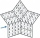Determine all pairs (m, n) of natural numbers for which is true: m s (n) = n s (m) = 70, where s (a) denotes the digit sum of the natural number a.
• Two cars on ring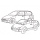There were two cars on the round track (ring) in the adjacent tracks, the first car in the inner track, the second in the outer track. Both cars started at the same time from one starting track. The first toy car drove every four laps simultaneously as th
• The largest numberFind the largest integer such that: 1. No figures is not repeat, 2. multiplication of every two digits is odd, 3. addition all digits is odd.
• Clubhouse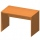There were only chairs and table in the clubhouse. Each chair had four legs, and the table was triple. Scouts came to the clubhouse. Everyone sat on their chair, two chairs were left unoccupied, and the number of legs in the room was 101. How many chairs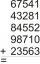Fill letters instead of digits so the indicated sum (equal letters represent equal digits). What number is hidden under the letter J? A A H A H O A H O J -------------------------- 4 3 2 1
• Beet campaign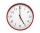The beet's field is being harvested. Beets are transported to 3 plants, 3 trucks. The first car returns to the field 3 times an hour, the second car after 30 minutes, and the third car 5 times an hour. All cars left at 8 in the morning. How many cars do w
• David numberJana and David train the addition of the decimal numbers so that each of them will write a single number and these two numbers then add up. The last example was 11.11. David's number had the same number of digits before the decimal point, the Jane's numbe
• Z9-I-4Kate thought a five-digit integer. She wrote the sum of this number and its half at the first line to the workbook. On the second line wrote a total of this number and its one fifth. On the third row, she wrote a sum of this number and its one nines. Fina
• Twos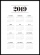Vojta started writing the number of this year 2019202020192020 into the workbook. .. And so he kept going. When he wrote 2020 digits, he no longer enjoyed it. How many twos did he write?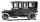Car started at 11:40 at speed 54 km/h from A and to B arrived at 12:20. Determine the distance A and B.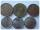Small Petra save money. Grandfather give her 10 crowns. Petra asked his older sister let her calculate how much he has saved up. Sister told her that he has less than 30 crowns. If the sum is divided by three left one crown. If the sum is divided by the f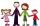Four families were on a joint trip. In the first family, there were three siblings, namely Alica, Betka and Cyril. In the second family were four siblings, namely David, Erik, Filip and Gabika. In the third family, there were two siblings, namely Hugo andThe self-counting machine works exactly like a calculator. The innkeeper wanted to add several three-digit natural numbers on his own. On the first attempt, he got the result in 2224. To check, he added these numbers again and he got 2198. Therefore, he a